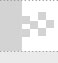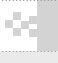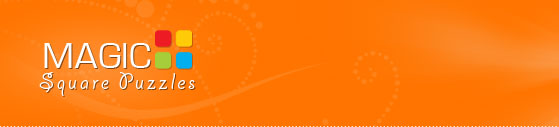Welcome, Guest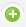Register |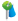Forgot Password |
 Sir Thomas Browne “God is a skilled Geometrician. ”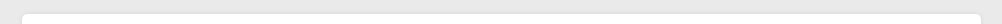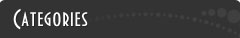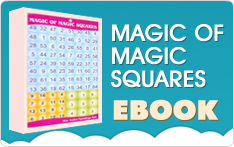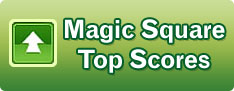Winner competition is underway now. Participate in this campaign and improve your mental mathematical skills.More Puzzle Games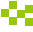Magic Square Puzzles  4x4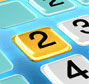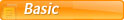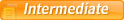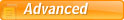Magic Square Puzzles  8x8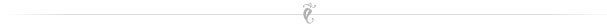Magic Square Puzzle

# Magic puzzle magic Square, Online Sudoku Games, Online Puzzle Games, Free Online Games, Maths Game, Brain Games, Puzzle Games, Magic Square Puzzles, brain puzzles, puzzles, mathematics, mathematics puzzle, math puzzle, Number puzzle, logic puzzle, arithmetic puzzMagic Square Puzzles are new kind of Puzzles. These Puzzles involve both Arithmetic and Logic.

In contrast, the Sudoku is just a logic puzzle. But the Magic Square Puzzle would improve your number-crunching skills as well as logical reasoning skills.

What is a magic square?

A magic square is an arrangement of numbers from n to n2 arranged so that no two numbers repeat, and all the rows, columns, and diagonals give the same total, which is called the magic sum.

 8 1 6 3 5 7 4 9 2

If you add all the rows, columns, and two diagonals, you would get the same total of 15. No number repeats in this magic square, and the magic sum is 15.

This is an example of the 3x3 magic square, and we also know that the 3x3 magic square is odd.

We will see one example for the even order magic square.

 9 16 5 4 7 2 11 14 12 13 8 1 6 3 10 15

This is an example of the Even order magic square. That, too, a doubly even order magic square.
The numbers that we have used are from 1 to 16, each only once such that all the 4 rows, 4 columns, and two  diagonals give the same magic sum of 34.

## The Magic Squares are of two types

1.Odd order Magic Squares and

2. Even order Magic Squares.

The Even order Magic Squares are again divided into two different types called the

1. Singly Even order magic squares

2. Doubly Even order Magic Squares.

The examples of the Singly even order magic squares are 6x6, 10x10, etc.

The numbers 6, 10, etc., are though even numbers are divisible by 2 but not divisible by 4.

The Doubly, Even order magic squares, are 4x4, 8x8, 12x12, etc. The numbers 4,8,12 etc., are divisible by 2 as well as by 4.

Here we will be dealing with only the Doubly Even order magic squares and that too 8x8 magic squares.